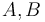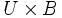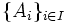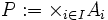# Product topology

## Contents

View a complete list of basic definitions in topology

## Definition for two spaces

Let$A,B$ be topological spaces. Then, we can consider the set$A \times B$: the Cartesian product of$A$ and$B$, which is the set of ordered pairs$(a,b)$ where$a \in A$ and$b \in B$. The product topology on$A \times B$ is defined in the following equivalent ways:

1. It is a topology with basis given by open rectangles in$A \times B$, viz., sets of the form$U \times V$ where$U$ is open in$A$ and$V$ is open in$B$.
2. Suppose we choose a basis for$A$ and a basis for$B$. Then, it is a topology with basis given by basis rectangles in$A \times B$, viz., sets of the form$U \times V$, where$U$ is a basis element of$A$ and$V$ is a basis element of$B$
3. It is a topology with subbasis given by open cylinders in$A \times B$, viz., sets of the form$A \times V$ where$V$ is open in$B$, or of the form$U \times B$ where$U$ is open in$A$.
4. It is the coarsest topology on$A \times B$ for which the projection maps to$A$ and$B$ are both continuous.

## Definition for an arbitrary family of spaces

Let$I$ be an indexing set and$\{ A_i \}_{i \in I}$ be a family of topological spaces. Consider the set:$P := \times_{i \in I} A_i$

viz., the Cartesian product of all the$A_i$s. The product topology on$P$ is given in the following equivalent ways:

1. It is a topology with basis given by subsets of the form$\times_{i \in I} U_i$, where$U_i$ are open subsets of$A_i$, and for all but finitely many$i$,$U_i = A_i$.
2. Suppose we choose a basis for each$A_i$. Then, it is a topology with basis given by subsets of the form$\times_{i \in I} U_i$, where$U_i$ are basis elements of$A_i$, and for all but finitely many$i$,$U_i = A_i$.
3. It is a topology with subbasis given by open cylinders: subsets of the form$\times_{i \in I} U_i$, where all the$U_i$s are open subsets of$A_i$, and at most one$U_i$ is a proper subset of the corresponding$A_i$
4. It is the coarsest topology on$P$ so that the projection maps to each of the$A_i$ is continuous.

## Related notions

Box topology is another topology on the Cartesian product of topological spaces, where the basis is all open boxes or open rectangles (i.e., we don't have the all but finitely many condition). For products of finitely many topological spaces, the box topology coincides with the product topology; in general, it is a finer topology.

Properties of topological spaces that are closed under taking products with the product topology, are listed in Category:Properties of topological spaces closed under products. Those properties that are closed under taking products of finitely many spaces, are listed in Category:Properties of topological spaces closed under finite products.